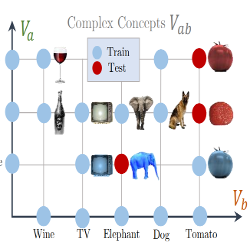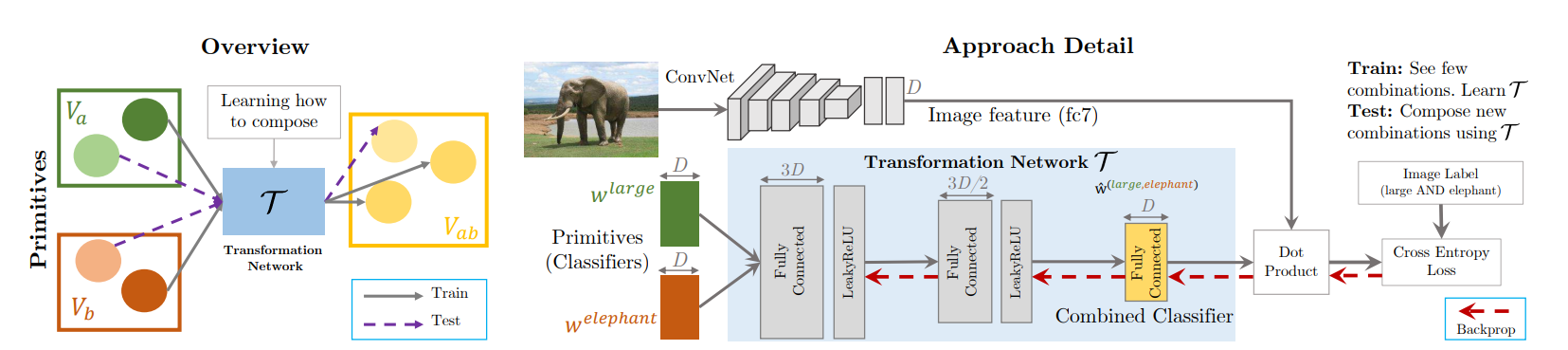Misra et al., CVPR, 2017

# From Red Wine to Red Tomato: Composition with ContextIn this paper, the authors tackle the problem of learning classifiers of visual concepts that can also adapt to new concept compositions at test time. The main difficulty is that visual concept can different depending on the context, i.e., depending on the concepts they are combined with. For instance the red in "red tomato" is different from the one in "red wine". This work emphasizes the notion of visual concepts as composition units, rather than the usual paradigm of directly learning from large exhaustive datasets.
• Pros (+): Purely visual (no extra linguistic/semantic source).
• Cons (-): Results are only reported on unseen combinations, hard to judge the base performance of the model.

## Proposed model

The main idea of the proposed method is to learn how to composite visual concepts (here: object categories and attributes) at the feature-level. Each visual concept $c$ is represented by a linear classifier $\phi_c: x \mapsto\ x \cdot w_c$ that has been trained to recognize it. The model then learns to compose such linear classifier by feeding them to a Transformation Network, $T$, which in turn outputs another classifier, which should capture the composition of the two input concepts.Figure: Overview of the Transformation Network. At training time, we assume access to a limited set of combinations of the primitives, each modeled by a linear classifier. The transformation network takes these classifiers as input and composes them to produce a classifier for their combination.

The input concepts are split in two categories (e.g., objects and attributes) which can be combined. For each of them, a linear binary classifier (SVM) is trained to detect the concept, based on some pre-trained feature extractor $\phi$. The goal is to learn the composition operation, which could be applied to even new combinations of the concepts, unseen during training. $T$ is parametrized as a 3 layer Multi-Layer Perceptron and trained with the following loss:

\begin{align} \mathcal{L}(x, a, b) = \ell(\text{sigmoid} ( T(w_a, w_b) \cdot \phi(x)), y) \end{align}

where $\ell$ is a standard binary classification loss (here, cross-entropy). $y$ is the label of the image $x$ for the considered task and should be 1 if and only if the image contains the complex context $(a, b)$.

## Experiments

The feature extractor used in the experiments is a VGG-M-1024 network pretrained on ImageNet. It is kept fixed and not re-trained, only the Transformation Network and linear classifiers are. Experiments are performed on the MITStates dataset and evaluated in terms of predicting (top-k accuracy and mAP) the presence or absence of unseen combinations of (object, attribute) pairs. The model is compared against the following baselines, using the same pre-trained backbone feature extractor:

• Individual: Only uses one of the modalities, e.g., $p(ab) = \max(p(a), p(b))$.
• Visual product: Classify complex concepts under independence assumption, $p(ab) = p(a) p(b)$.
• Label Embeddings: [1, 2] The transformation network is learned on word embeddings of the visual concepts as inputs.

Interestingly the Individual-Obj baseline that considers only the object category performs quite well in terms of average precision. This suggest the object category is more significant than the attribute prediction. Finally, the proposed model outperforms the baselines but still produces poor overall performance in terms of classification accuracy, so it is not clear how much is really due to generalization to new concept combinations. Ablation and qualitative experiments do show that the model learns some meaningful information about composition, however the gain seems to depend a lot on the actual object and attribute combined.

## References

•  Predicting Deep Zero-shot Convolutional Neural Networks using Textual Descriptions, Ba et al, CVPR, 2015
•  Zero-Shot Learning using purely Textual Descriptions, Everingham et al, ICCV 2013